# Quiz 2: Fundamental Economic Concepts

Business

In order to keep on charging differentiated prices to customer in the same class of service, American Airlines should resolve following issues: i) It should try to create apparent value for the customers segmented into different classes. ii) It should plan and allocate the airline capacity in advance of the arrival of the customers so as to minimize unsold inventory. iii) It should partition the demand so as to smoothen the demand. iv) It should offer price incentives so as to utilize the scarce resources more efficiently. v) It should creatively use the off-peak capacity.

The demand function for hybrid gasoline-electric vehicle- Toyota Prius-is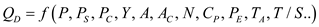where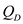is the quantity demanded of Toyota Prius, P is the price of Toyota Prius,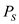is the price of substitute goods such as Chevy Volt,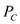is the price of complementary goods such as gasoline, Y is the income of consumers, A is the advertising and promotion expenditures by Toyota,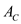is the competitors' advertising and promotion expenditures, N is the size of the potential target market,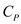is the consumer tastes and preferences for a "greener" form of transportation,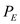is the expected future price of hybrid autos,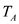is the purchase adjustment time period and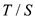is the taxes or subsidies on hybrid autos. An increase in the price of Toyota Prius would decline the quantity demanded. An increase in the price of Chevy Volt (a substitute) would increase the demand for Toyota Prius as it is relatively less expensive. Demand curve would shift rightwards and equilibrium price would increase. An increase in the price of gasoline (a complement) would decrease the demand for Toyota Prius as it increases the cost of driving. Demand curve would shift leftwards and equilibrium price would decline. An increase in the income of the consumers would increase the demand for Toyota Prius as they have more money to spend. Demand curve would shift rightwards and equilibrium price would increase. An increase in the advertising expenditure by Toyota would increase the demand for product as it is more popular among the consumers. Demand curve would shift rightwards and equilibrium price would increase. An increase in the advertising expenditure by Chevy Volt would decrease the demand for Toyota Prius as it is comparatively less popular among the consumers. Demand curve would shift leftwards and equilibrium price would decrease. An increase in the advertising expenditure by Toyota would increase the demand for product as it is more popular among the consumers. Demand curve would shift rightwards and equilibrium price would increase. An increase in the target population is likely to increase the demand for Toyota Prius as there are more people who know the existence of the product in the market. Demand curve would shift rightwards and equilibrium price would increase. An increase in the preferences of consumers for greener form of transportation would increase the demand for Toyota Prius. Demand curve would shift rightwards and equilibrium price would increase. An expectation of price increase in future would increase the demand for Toyota Prius. Demand curve would shift rightwards and equilibrium price would increase. An increase in the adjustment time would increase the demand for Toyota Prius. Demand curve would shift rightwards and equilibrium price would increase. Imposition of taxes on the purchase of Toyota Prius increases its price and thus, would decrease its demand. Demand curve would shift leftwards and equilibrium price would fall. Note: Supply and other determinants of demand remain unchanged for any given change in a particular determinant. The supply function for hybrid gasoline-electric vehicle-Toyota Prius- is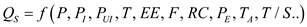where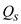is the quantity supplied of Toyota Prius, P is the price of Toyota Prius,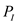is the price of inputs such as sheet metal,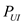is the price of unused substitute inputs such as fiberglass, T is the technological improvement, EE is the entry or exit of other auto sellers, F is the accidental supply interruptions from fires, floods, etc., RC is the costs of regulatory compliance,is the expected future price of hybrid autos,is the purchase adjustment time period andis the taxes or subsidies on hybrid autos. An increase in the price of Toyota Prius would increase the quantity supplied. An increase in the price of steel metal (an input) would decrease the supply for Toyota Prius as the production becomes more costly. Supply curve would shift leftwards and equilibrium price would increase. An increase in the price of fiberglass (an unused substitute input) would decrease the supply. Supply curve would shift leftwards and equilibrium price would increase. Technological improvements would increase the supply as production is more efficient. Supply curve would shift rightwards and equilibrium price would fall. Entry of other sellers would increase the supply for Toyota Prius as there are more producers in the market. Supply curve would shift rightwards and equilibrium price would fall. Interruptions in supply due to accident would decrease the supply as the supplier would be left with lesser output. Supply curve would shift leftwards and equilibrium price would increase. An increase in the cost of regulatory compliance would increase the cost of production and thus, supply would decline. Supply curve would shift leftwards and equilibrium price would increase. An expectation of price increase in future would decrease the supply for Toyota Prius. Supply curve would shift leftwards and equilibrium price would increase. Greater adjustment time would increase the supply for Toyota Prius. Supply curve would shift rightwards and equilibrium price would fall. Imposition of taxes on the sales of Toyota Prius reduces the price received by the producer. This would decrease its supply. Supply curve would shift leftwards and equilibrium price would increase. Note: Demand and other determinants of supply remain unchanged for any given change in a particular determinant.

Yes the revenue management techniques applied in Airline industry can also be successfully applied in industries like trucking, health care industry and hotel industry. This is because all require scheduling of capacity before the demand as they all face the same issue faced by American Airlines and are part of the service industry.